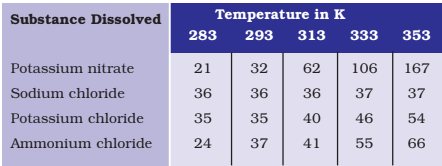Q

# Pragya tested the solubility of three different substances at different temperatures and collected the data as given below (results are given in the following table, as grams of substance dissolved in 100 grams of water to form a saturated solution) (a)

Q 3.     Pragya tested the solubility of three different substances at different temperatures and collected the data as given below (results are given in the following table, as grams of a substance dissolved in 100 grams of water to form a saturated solution).(a)       What mass of potassium nitrate would be needed to produce a saturated solution of potassium nitrate in 50 grams of water at 313 K?

Views

We have,
Mass of potassium nitrate = 62g in 100 g of water

Therefore, according to question,
Mass of potassium nitrate in 50 g of water at 313K

Exams
Articles
Questions